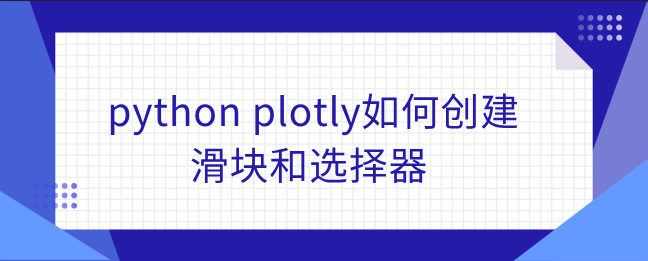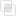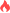python plotly如何创建滑块和选择器

Python plotly2021-08-20 18:04:12954浏览 · 0收藏 · 0评论1、范围滑块是plotly中自定义范围类型的输入控制器。

2、范围选择器是选择图表中显示范围的工具。该按钮用于在图表中选择预配置范围。

```import plotly.graph_objects as px
import pandas as pd

# 读取数据库

plot = px.Figure(data=[px.Scatter(
y=data['tip'],
mode='lines',)
])

plot.update_layout(
xaxis=dict(
rangeselector=dict(
buttons=list([
dict(count=1,
step="day",
stepmode="backward"),
])
),
rangeslider=dict(
visible=True
),
)
)

plot.show()```python中Laplacian算子如何使用python中Laplacian算子是什么python列表遍历的形式python输入函数input的使用python print两种输出形式python控制语句的两大分类python编写程序的常见错误python用户如何自定义异常python thread模块创建线程python threading实现线程的过程python threading线程同步如何实现python聚类算法是什么python聚类算法如何选择python中plotly添加交互的方法css超链接是什么304

mysql InnoDB的崩溃恢复过程278

php类自动加载失败的解决办法263